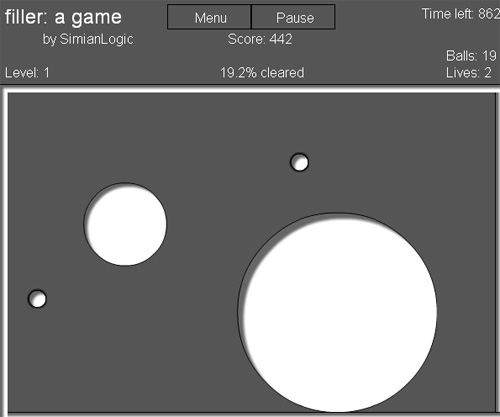# Basic Filler engine with Box2D – part 1

Do you remember Filler?In Filler, your goal is simple: fill 2/3 of the level. To create a filler ball, press down. It will grow until you release the mouse button, it hits another filler ball, or a bouncing ball runs into it.

I am showing you the first part, the creation of the filler ball by pressing down the mouse, with no collision detection while you are creating the ball.

The code is mainly taken from Drawing circles on the fly in Box2DfleshMaker version, but uses movieclips for the balls (so I removed the debug draw) and has a different way of creating circles on the fly.

This is the script:

```package {
import flash.display.Sprite;
import flash.events.Event;
import flash.events.MouseEvent;

import Box2D.Dynamics.*;
import Box2D.Collision.*;
import Box2D.Collision.Shapes.*;
import Box2D.Common.Math.*;

public class colorballz extends Sprite {
public var m_dbgSprite;
public var m_world:b2World;
public var m_phys_scale:Number = 30;
public var m_timestep:Number = 1.0/30.0;
public var m_iterations:Number = 10.0;

//initial box coordinates when we first press mouse down
public var initX:Number = 0.0;
public var initY:Number = 0.0;
public var drawing:Boolean = false;
public var b:b2Body;

//
public var the_ball:ball;
public function colorballz() {
/*
A Box2D world needs three parameters: a b2AABB, gravity
and a Booleand deciding whether or not to let bodies sleep
when they are not being simulated.
This saves CPU so should always be left on :)
*/

var gravity:b2Vec2 = new b2Vec2(0,9.8);
var worldAABB:b2AABB = new b2AABB();
worldAABB.lowerBound.Set(-1000,-1000);
worldAABB.upperBound.Set(1000,1000);
m_world = new b2World(worldAABB,gravity,true);

}
public function mousePressed(e:MouseEvent) {
//Store initial X and Y position
initX = e.localX;
initY = e.localY;
the_ball = new ball();
the_ball.x = mouseX;
the_ball.y = mouseY;
the_ball.width = 1;
the_ball.height = 1;
drawing = true;
}
public function mouseMoved(e:MouseEvent) {
if (drawing) {
the_ball.x = mouseX;
the_ball.y = mouseY;
}
}
public function mouseReleased(e:MouseEvent) {
drawing = false;
}
var bd:b2BodyDef = new b2BodyDef();
var cd:b2CircleDef = new b2CircleDef();
// area is the % of the entire stage filled by the circle
// the entire stage area is 500*500 = 250000 pixels
cd.density = 2;
cd.restitution = 0.7;
cd.friction = 2;
bd.position.Set(_x/m_phys_scale, _y/ m_phys_scale);
bd.userData = ballclip;
b = m_world.CreateBody(bd);
b.CreateShape(cd);
b.SetMassFromShapes();
}
var bodyDef:b2BodyDef = new b2BodyDef();
bodyDef.position.Set(_x,_y);
var boxDef:b2PolygonDef = new b2PolygonDef();
boxDef.SetAsBox(_halfwidth,_halfheight);
boxDef.density = 0.0;
var body:b2Body = m_world.CreateBody(bodyDef);
body.CreateShape(boxDef);
body.SetMassFromShapes();
}
public function Update(e:Event) {
//We need to do this to simulate physics
if (drawing) {
the_ball.width+=2;
the_ball.height+= 2;
}
m_world.Step(m_timestep,m_iterations);
for (var bb:b2Body=m_world.m_bodyList; bb; bb=bb.m_next) {
if (bb.m_userData is Sprite) {
bb.m_userData.x=bb.GetPosition().x * 30;
bb.m_userData.y=bb.GetPosition().y * 30;
bb.m_userData.rotation=bb.GetAngle() * 180 / Math.PI;
}
}
}
}
}
```

There are some inline comments, and there is nothing that new in the code.

Here it is the result:

Click and hold to make the ball grow, release to create it.

And this is the source.

Next step we will see something new: how to make some objects to be affected by gravity and some other not to be affected.

214 GAME PROTOTYPES EXPLAINED WITH SOURCE CODE
// 1+2=3
// 10000000
// 2 Cars
// 2048
// Avoider
// Ballz
// Block it
// Blockage
// Bloons
// Boids
// Bombuzal
// Breakout
// Bricks
// Columns
// CubesOut
// Dots
// DROP'd
// Dudeski
// Eskiv
// Filler
// Fling
// Globe
// HookPod
// Hundreds
// InkTd
// Iromeku
// Lumines
// Magick
// MagOrMin
// Maze
// Memdot
// Nano War
// Nodes
// o:anquan
// Ononmin
// Pacco
// Phyballs
// Platform
// Poker
// Pool
// Poux
// Pudi
// qomp
// Racing
// Renju
// SameGame
// Security
// Sling
// Slingy
// Sokoban
// Splitter
// Sproing
// Stack
// Stringy
// Sudoku
// Tetris
// Threes
// Toony
// Turn
// TwinSpin
// vvvvvv
// Wordle
// Worms
// Yanga
// Zhed
// zNumbers